# quants-simple-compound-interest-quiz3Simple & Compound Interest Quiz-3

Maths is an important subject in CLAT,DULLB & Other Law Exams. In any of law exam, Maths carries weightage of 20 -25 % of questions. With focused practice good marks can be fetched from this section. These questions are very important in achieving your success in CLAT, DULLB and Other Law Exams..

Q1. The simple interest on Rs. 500 at 6% per annum from May 3rd to July 15th in the same year is

•  Rs. 9
•  Rs. 6
•  Rs. 4
•  None of these
Solution
Time from May 3rd to July 15th
= 28 days of May + 30 days of June and 15 days of July
= 73 days = 1/5 years

I =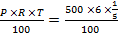= Rs.6

Q2.A sum of money at simple interest comes four times in 24 years. The rate Percent of interest per annum is
•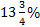•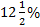•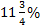•  None of these
Solution
b): We have, n = 4 and T = 24 years
Rate of interest
=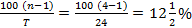Q3.What should be the least number of years in which the simple interest on Rs 2600 at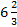% will be exact number of rupees?
•  2
•  3
•  4
•  5
Solution
(b): SI =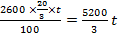T has to be a multiple of 3

Q4. A sum of money doubles itself at CI in 15 year. In how many years will it become eight times?

•  45
•  50
•  55
•  60
Solution
(a): A = 2P = P (1 + r)15 r = 21/15 - 1
A = 8P = P(1 + 215 - 1)t 8 = 23 = 21/15

T = 45

Q5.A certain sum amounts to Rs. 7300 in 2 years & to Rs. 8575 in 3 years compounded annually. Find the sum
•  Rs. 5400
•  Rs. 5290
•  Rs. 5600
•  Rs. 5600
Solution
A = P (1 + r)t; 7300 = P (1 + r)2

8575 = P (I + r)3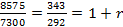P = 7300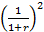=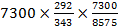= 5290
Q6. The amount of Rs 7500 at compound interest at 4% per annum for 2 years is.

•  Rs. 7800
•  Rs. 8100
• Rs. 8112
•  Rs. 8082
Solution
(c): A = 7500 (1 + .04)2 = 7500 x 1.0816 = 8112

Q7. 27. If the SI on a sum of money at 5% per annum for 3 years is Rs. 1200, the CI on the same sum for the same period & rate is?
•  Rs. 1260
•  Rs. 1261
•  Rs. 1264
•  Rs. 1265
Solution
(b): SI = 1200 =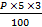P = 8000
A = 8000(1.05)3 =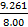(8000) = 9261

CI = 9261 – 8000 = 1261

Q8. The difference between the CI & SI on a sum of money for 2 years at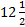per year is Rs 150. The sum is

•  Rs. 9000
•  Rs. 9200
•  Rs. 9500
•  Rs. 9600
Solution
d): SI & Cl for 1st yr
150 =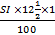; SI = 600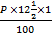P = 9600

Q9. If the difference between CI, compounded half early & the SI on a sum at 10% per annum for one year is Rs 25. The sum is.

•  Rs. 9000
•  Rs. 9500
•  Rs.10000
•  Rs. 10500
Solution
(c): Interest for half year = 5%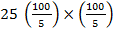= 10,000

Q10. If the CI on a certain sum at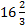% for 3 years is Rs 1270, the SI on the same sum at the same rate & for the same period is

• Rs. 1200
• Rs. 1165
• Rs. 1080
• Rs. 1220
Solution
c): Cl = A - P = P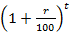– P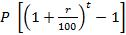= 1270 P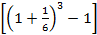= 1270
P =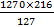P = 2160

This post first appeared on Clear Law Entrance - Clear Exam, please read the originial post: here

# Share the post

quants-simple-compound-interest-quiz3

×